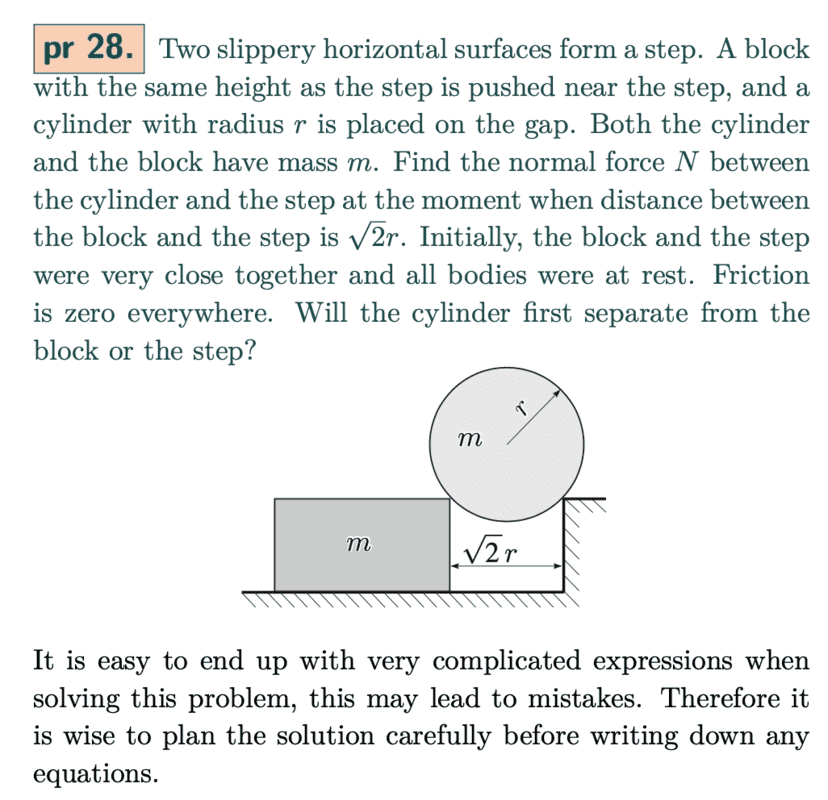# Conservation of energy in rotating bodies

• phantomvommand
In summary, the conservation of energy equation is basically GPE is converted to KE of block and KE of cylinder. The KE of the cylinder is 1/2mv^2, where m is its mass and v is the velocity of its COM (which is the centre of cylinder). However, I viewed the cylinder as rotating about the point of contact with the step, and thus thought that its KE was 1/2Iw^2, where I = 3/2mr^2 (by parallel axis theorem), and rw = v, where v is the velocity of the centre of mass.

#### phantomvommandThe conservation of energy equation is basically GPE is converted to KE of block and KE of cylinder.

To get the correct answer, the KE of the cylinder is 1/2mv^2, where m is its mass and v is the velocity of its COM (which is the centre of cylinder).
However, I viewed the cylinder as rotating about the point of contact with the step, and thus thought that its KE was 1/2Iw^2, where I = 3/2mr^2 (by parallel axis theorem), and rw = v, where v is the velocity of the centre of mass.

Why is my calculation of KE for the cylinder wrong?

This problem can be found in Jaan Kalda's Mechanics problems, or in SS Krotov's Aptitude Test Problems.

phantomvommand said:
Summary:: Why is the kinetic energy I ascribe to the cylinder in the following question wrong?

Why is my calculation of KE for the cylinder wrong?
Because the cylinder is not rotating.

phantomvommand said:
I viewed the cylinder as rotating about the point of contact with the step

as they above say your work is wrong, but u have some ok intuition. cylinder not rotate about its mass centre, however the mass centre point do rotate in circular arc about the contact point on right (well, so long as it is still contact!). this you can use to advantage! say, mass centre of cylinder has rotate by ##\theta## about right point of contact. let ##v = |\underline{v}|## be speed of the mass centre. can first use the energy conserve$$2gr(1-\cos{\theta}) = v^2$$now, remember that mass centre move as if it were particle which is act upon by resultant force, so you now pretend mass centre is a particle, and resolve resultant of force toward right contact point (i.e. you calculate centripetal component):$$-N + mg\cos{\theta} + N_2 \sin{2\theta} = \frac{mv^2}{r}$$where ##N## is force of contact from right point, and ##N_2## force of contact from left point. now you must do examine cases when ##N = 0## and ##N_2 = 0## respective, i.e. set each to ##0## in turn and use two equation to eliminate ##v##, and find ##\theta_{\text{max}}## for each. then you can tell, which lose contact first.

see - much more easy than do use lot of messy coordinate and difficult equation, yes ;)

## 1. What is conservation of energy in rotating bodies?

Conservation of energy in rotating bodies refers to the principle that the total energy of a system remains constant as long as there are no external forces acting on it. This means that the energy of a rotating body will not change unless there is an external torque applied to it.

## 2. How does conservation of energy apply to rotating bodies?

Conservation of energy applies to rotating bodies through the concept of angular momentum. Since angular momentum is conserved in a closed system, any changes in rotational energy must be offset by an equal and opposite change in the moment of inertia or angular velocity of the body.

## 3. What are some examples of conservation of energy in rotating bodies?

A common example of conservation of energy in rotating bodies is a spinning top. As the top loses energy due to friction, its rate of rotation decreases, but the moment of inertia also decreases to maintain conservation of energy. Another example is a planet orbiting a star, where the planet's kinetic energy and potential energy balance each other out to maintain a constant total energy.

## 4. Can conservation of energy be violated in rotating bodies?

No, conservation of energy cannot be violated in rotating bodies as long as the system is closed and there are no external forces acting on it. Any changes in energy must be balanced out by changes in other factors such as moment of inertia or angular velocity to maintain the total energy of the system.

## 5. How is conservation of energy in rotating bodies related to the laws of thermodynamics?

Conservation of energy in rotating bodies is directly related to the first law of thermodynamics, which states that energy cannot be created or destroyed, only transformed from one form to another. In rotating bodies, energy may change from kinetic to potential or vice versa, but the total energy of the system remains constant.